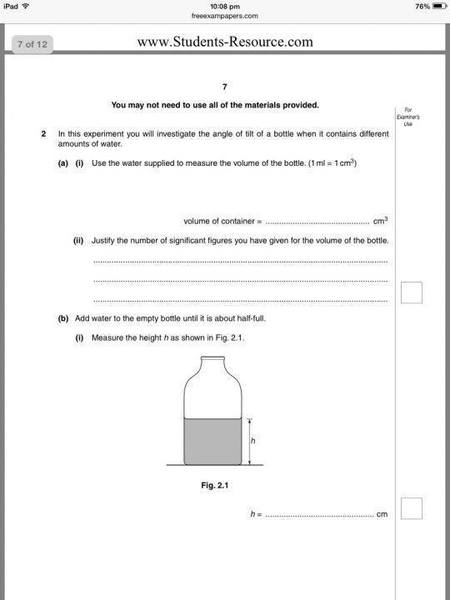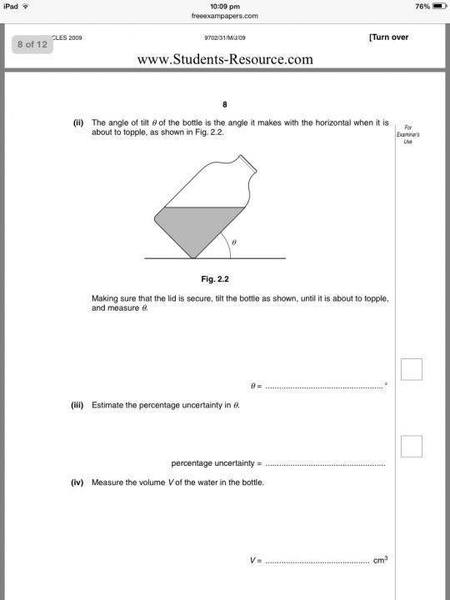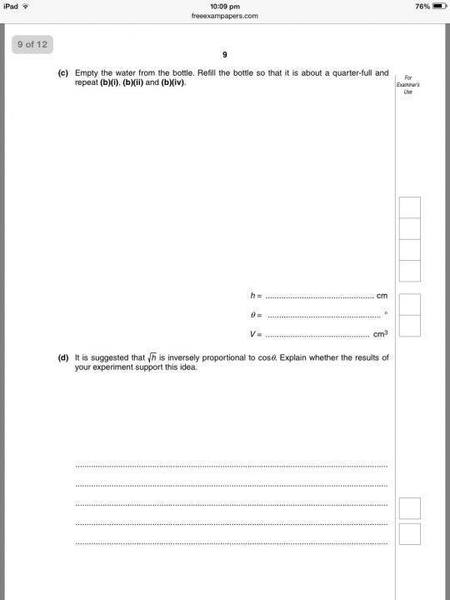# Physics Practical: Check proportionality

## Homework StatementFor part d), how to show that they are inversely proportional to each other ? The mark scheme states:

"Correct calculation to check inverse proportionality. √h x cosϑ = k. Sensible comments relating to calculations to within 20% or their own value and suggested relation."

What do they mean by 20%?

## Homework Equations

Percentage uncertainty = (uncertainty/measured value) x100%

## The Attempt at a Solution

My values are,

√h = (2.95±0.1) cm, cos θ = 0.50. k1 = 2.95 x 0.50 = 1.475
√h = (2.30±0.1) cm, cos θ = 0.64, k2 = 2.30 x 0.64 = 1.472

I calculated the percentage difference of k,
((1.475-1.472) /1.472 ) x 100% = 0.2%

Then I calculated the uncertainty of √h = (0.1 / 2.30) x 100% = 4.3%

This is the method I followed from a textbook, so what does the 20% in the mark scheme represents ? Hope you all understand what I'm doing here..

mfb
Mentor
The square root of a height should not have centimeters as units.

A 20% uncertainty which the quoted text might suggest would be very large.

I don't like the way the problem is set up. With just those two measurements, it is not reasonable to draw any conclusions about the relation between h and θ.

Fun exercise: calculate the height h which allows tilting the bottle by 90 degrees without tipping over. Is this realistic?

The square root of a height should not have centimeters as units.

A 20% uncertainty which the quoted text might suggest would be very large.

I don't like the way the problem is set up. With just those two measurements, it is not reasonable to draw any conclusions about the relation between h and θ.

Fun exercise: calculate the height h which allows tilting the bottle by 90 degrees without tipping over. Is this realistic?

Oh yeah, was not aware, sorry.

Hmm.. what do you mean tilting the bottle by 90 degrees?

mfb
Mentor
Which height h corresponds to θ=0? Is that realistic?
What happens if you reduce h a bit more?